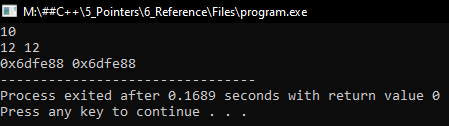# Reference in C++

## Reference in C++ with Examples:

In this article, I am going to discuss Reference in C++ Language with examples. Please read our previous articles, where we discussed the Disadvantages of using Pointers in C++ with examples. Reference is one of the powerful features of C++. This feature is not there in any other language. Let us see what it is.

##### What is Reference in C++?

Let is understand reference with an example.

int main(){
int x = 10;
int &y = x;
}

Here in the main function, we have declared a variable ‘x’ and assigned it to ‘10’. Memory is allocated for ‘x’ as follows.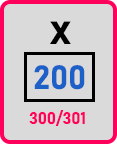On the next line, we have declared another variable ‘y’ with the prefix ‘&’ and assigned it to ‘x’. If we write only ‘y’ then it’s a normal variable. So, for now, any memory will be allocated for ‘y’? No, this looks a little different. If we add ‘*’ at the place of ‘&’ then ‘y’ will be a pointer.

So here ‘&’ means reference. And whenever we declare a reference, we must initialize it that time only. So, we assign it to ‘x’. So, what is Reference?

Reference is nothing but a nickname of a variable or alias of a variable. So ‘y’ is just another name of ‘x’. The same memory ‘200’ will be shared to ‘y’ means ‘x’ and ‘y’ are the same thing but different names.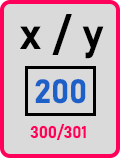So ‘y’ is an alias or nickname of variable ‘x’.

##### Why do you need References in C++?

We don’t need this inside the same function. We will discuss this in the next article. Now ‘200/201’ can be called with the name ‘x’ and can be called with the name ‘y’ also. If we say ‘x++’ then the value becomes ‘11’. Same way if we say ‘y++’ then the value becomes ‘12’. Both ‘x’ and ‘y’ are accessing the same memory.

If we write ‘cout << x’ then we will get the value ‘12’ and if we write ‘cout << y’ then also we will get the value ‘12’. So definitely it is not required in the same function. Just we are learning what is the reference.

int a;

a = x;

x = 22;

Here we have declared one more variable ‘a’ and then we have assigned it to ‘x’. On the next line, we assigned ‘x’ to ‘22’. Let us see what is the difference between these two statements.

In ‘a = x’, ‘x’ is used on the right-hand side of an assignment, and in ‘x = 22’, ‘x’ is used on the left-hand side of the assignment. So ‘a = x’, ‘x’ is right-hand side so we say it is R-value and ‘x = 22’, ‘x’ is on the left-hand side so we call it as L value. Now let us understand these two things.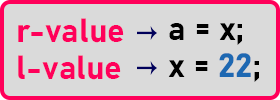R-value means, whatever is there in ‘x’ has to be stored in ‘a’. ‘x’ is 12, so 12 will be stored in ‘a’. So, when we write X it means the value of the video but X so that the value is stored here. So, value is like a literal constant value. R-value is data.

In L value we are saying ‘x’ assign 22, we want 22 to be stored in ‘x’. So, 22 should be stored there erasing whatever was there. It will overwrite and ‘22’ will be stored there. We can say that,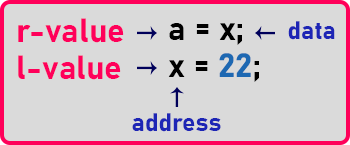So ‘x’ is data when you take a variable on the right-hand side. And if we write it on the left-hand side it means its address.

In case of reference when you have written ‘&y’ assign ‘x’ so ‘x’ is written on the right-hand side. What is given in the name of ‘y’? That same address will give for ‘y’ also. So, it means here ‘x’ is the L value of ‘x’. Now one more important thing that is ‘x’ is occupying supposed 2 bytes as it is the type of integer. Then how much memory ‘y’ is occupying? ‘y’ is not occupying any memory. That is one more important thing about reference, the reference doesn’t consume any memory at all. It is just like your brother has bought a car and that same car belongs to you also.

So, whether you call ‘x’ or ‘y’, it’s the same thing. Now one more important thing about references is once you have declared and initialized reference you cannot make it as a nickname for any other variable. Now it is meant for only x. You cannot write again ‘&y = a’. So ‘y’ cannot be referencing any other variable at all.

##### Reference Key Points:
1. Reference is an Alias of variable
2. It must be initialized when declared
3. It doesn’t take any memory
4. It cannot be modified to refer to another variable
5. The syntax for reference declaration is int &y=x
##### Program on Reference in C++:
```#include <iostream>
using namespace std;
int main()
{
int x = 10;
int &y = x;

cout << x << endl;
y++;
x++;

cout << x << " " << y << endl;
cout << &x << " " << &y;

return 0;
}
```
###### Output: